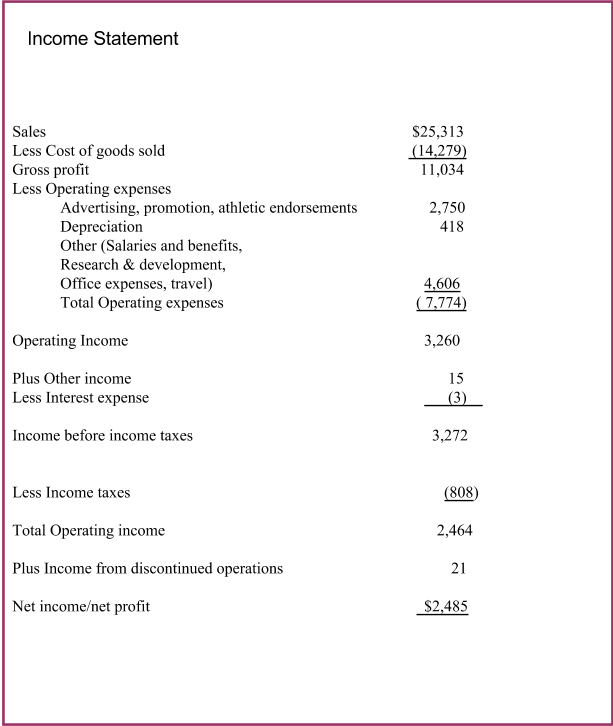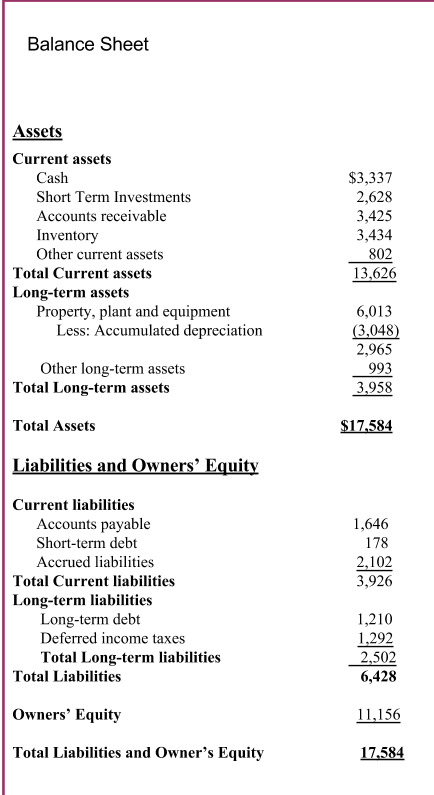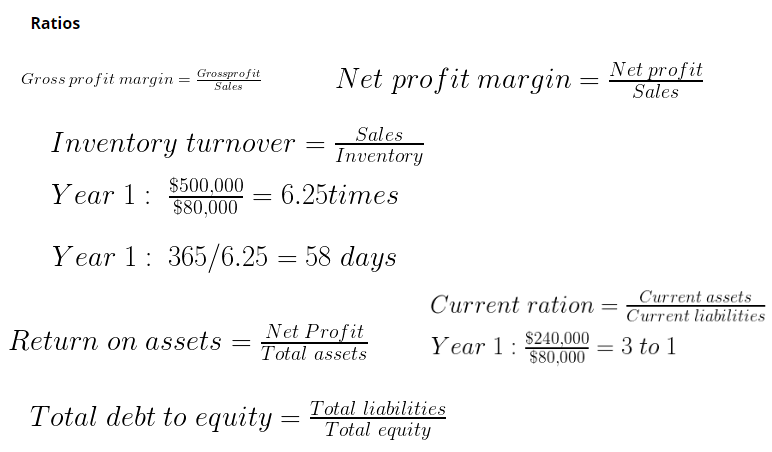Intro to Business Ch 12 Quiz - Print Student Copy
Email address *
1. Accounting information is only prepared for people within the company.
1 point
Accountants communicate the meaning of _____ information and work with individuals and organizations to help them use that information to deal with business problems.
1 point
Your _____ shows the amount of cash that you have coming in and going out.
1 point
Your _____ statement shows your revenues and expenses.
1 point
Assets like buildings and inventory would be shown on a company’s _________.
1 point
The accounting equation used for the balance sheet is assets = ________ + owner’s equity.
1 point
Your income statement has show that you are making money, but you want more. Which of the following are things you could do to increase your net profit?
1 point
Use these financial statements from Nike and ratios to answer the following questions.Use these financial statements from Nike and ratios to answer the following questions.Use these financial statements from Nike and ratios to answer the following questions.Calculate Nike’s gross profit margin.
1 point
Your answer
Calculate Nike’s net profit margin. What does this ratio tell you (in general, not specifically for Nike)? Two points.
2 points
Your answer
Calculate Nike’s inventory turnover. If the industry average is 60 days, how is Nike performing on this ratio? Explain your answer. Two points.
2 points
Your answer
Calculate Nike’s return on assets. If the industry average is 10%, would you be happy as an investor? Explain your answer. Two points.
2 points
Your answer
Calculate Nike’s current ratio.
1 point
Your answer
Calculate Nike’s total debt to equity. For every dollar of equity, Nike has ______ in debt. If the industry average is \$0.25 of debt for every \$1.00 of equity, will it be easy or hard for Nike to borrow money? Explain your answer. Two points.
2 points
Your answer
Which of the following are basic things every business person should do in order to be successful?
1 point
Never submit passwords through Google Forms.
This content is neither created nor endorsed by Google. Report Abuse - Terms of Service